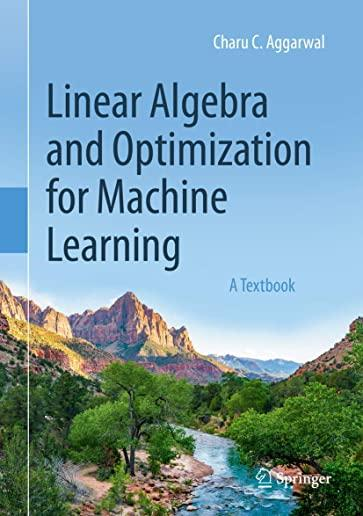### Linear Algebra and Optimization for Machine Learning: A Textbook### Huge savings for students

Each student receives a 50% discount off of most books in the HSG Book Store. During class, please ask the instructor about purchase details.
 List Price: \$69.99 Price: \$35.00 You Save: \$35.00
1

This textbook introduces linear algebra and optimization in the context of machine learning. Examples and exercises are provided throughout the book. A solution manual for the exercises at the end of each chapter is available to teaching instructors. This textbook targets graduate level students and professors in computer science, mathematics and data science. Advanced undergraduate students can also use this textbook. The chapters for this textbook are organized as follows:

1. Linear algebra and its applications: The chapters focus on the basics of linear algebra together with their common applications to singular value decomposition, matrix factorization, similarity matrices (kernel methods), and graph analysis. Numerous machine learning applications have been used as examples, such as spectral clustering, kernel-based classification, and outlier detection. The tight integration of linear algebra methods with examples from machine learning differentiates this book from generic volumes on linear algebra. The focus is clearly on the most relevant aspects of linear algebra for machine learning and to teach readers how to apply these concepts.

2. Optimization and its applications: Much of machine learning is posed as an optimization problem in which we try to maximize the accuracy of regression and classification models. The "parent problem" of optimization-centric machine learning is least-squares regression. Interestingly, this problem arises in both linear algebra and optimization, and is one of the key connecting problems of the two fields. Least-squares regression is also the starting point for support vector machines, logistic regression, and recommender systems. Furthermore, the methods for dimensionality reduction and matrix factorization also require the development of optimization methods. A general view of optimization in computational graphs is discussed together with its applications to back propagation in neural networks.

A frequent challenge faced by beginners in machine learning is the extensive background required in linear algebra and optimization. One problem is that the existing linear algebra and optimization courses are not specific to machine learning; therefore, one would typically have to complete more course material than is necessary to pick up machine learning. Furthermore, certain types of ideas and tricks from optimization and linear algebra recur more frequently in machine learning than other application-centric settings. Therefore, there is significant value in developing a view of linear algebra and optimization that is better suited to the specific perspective of machine learning.

Springer